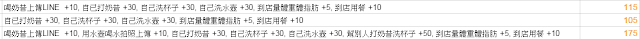## 2010年8月1日

### [Excel 技巧] 善用資料剖析來調整資料格式 (以 MS Excel 2007 為例)

( =IF(OR(ISBLANK(A2), EXACT(A2, "N/A")), "", SUBSTITUTE(MID(A2, 1, 3), ".", "")+1911) )

• Start Year: =IF(OR(ISBLANK(A2), EXACT(A2, "N/A")), "", SUBSTITUTE(MID(A2, 1, 3), ".", "")+1911)
• Start Month (TMP): =IF(OR(ISBLANK(A2),EXACT(A2,"N/A")),"",SUBSTITUTE(MID(A2,4,2),".",""))
• Start Day (TMP): =IF(OR(ISBLANK(A2),EXACT(A2,"N/A")),"",SUBSTITUTE(MID(A2, FIND(".", A2, 4)+1, 2), "~", ""))
• Start Month: =IF(OR(ISBLANK(A2),EXACT(A2,"N/A")),"",REPT(0, 2-LEN(N2))&N2)
• Start Day: =IF(OR(ISBLANK(A2),EXACT(A2,"N/A")),"",REPT(0, 2-LEN(O2))&O2)
• End Year: =IF(OR(ISBLANK(A2),EXACT(A2,"N/A")),"", SUBSTITUTE(MID(A2, FIND("~", A2)+1, 3), ".", "")+1911)
• End Month (TMP): =IF(OR(ISBLANK(A2),EXACT(A2,"N/A")),"",SUBSTITUTE(MID(A2, FIND("~", A2)+4, 2), ".", ""))
• End Day (TMP): =IF(OR(ISBLANK(A2),EXACT(A2,"N/A")),"",SUBSTITUTE(RIGHT(A2, 2), ".", ""))
• End Month: =IF(OR(ISBLANK(A2),EXACT(A2,"N/A")),"",REPT(0, 2-LEN(S2))&S2)
• End Day: =IF(OR(ISBLANK(A2),EXACT(A2,"N/A")),"",REPT(0, 2-LEN(T2))&T2)